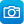# Search results

Year level
Resource type
Learning area

## Refine by topic

Main topic Specific topic Related topic
Listed under:  Area### Turn up the volume - unit of work

In this unit of work students explore and explain the connections between the surface area and volume of different shapes and how each attribute is measured.### TIMES Module 11: Measurement and Geometry: area, volume and surface area - teacher guide

This is a 15-page guide for teachers containing explanations of the derivation of formulas for the areas of parallelograms, trapeziums, rhombuses and kites. Formulas for the volumes and surface areas of prisms and cylinders are obtained. Applications of these formulas are given. A history of the development of these concepts ...### Giant hole: climb through a hole in a sheet of paper

This teaching resource outlines an activity for students to explore the relationship between the area and perimeter of a shape, by using a series of cuts in a piece of paper to create a hole large enough to step through. The resource includes detailed instructions for creating the cuts in the paper, an explanation of how ...### Measuring our coastline

How long is the Australian coastline? See Dr Derek Muller and Simon Pampena discussing the perimeter of the Australian coastline. Find out how the accuracy of that measurement depends on the length of the 'measuring stick' used. They discuss how a coastline is much like a fractal such as 'Koch's Snowflake'!### Mapping farmland: using area and trigonometry

In northern Queensland's Gulf region, some farmers use GPS mapping to help manage their extensive properties. Use this clip as a context for applying your understanding of area, in particular your understanding of conversion between square kilometres and hectares. Apply trigonometry and Pythagoras' theorem.### Drawing a floor plan

How do we know what a house will look like before it is built? Discover how house plans work by looking at the design of a house that Hugo's family is going to build. See how a floor plan shows the room layout. See drawings of what the house will look like from different views.### Calculating area: locust plagues

How many locusts in a plague? Find out just how big the threat of locusts can be and how farmers try to prevent the plagues from getting out of control. This clip provides context for a combination of area, area units and rate problems.### Working out the areas

Do you know how to work out the area of a square, a rectangle or a triangle? Learn the simple maths formulas needed from this video. What would be the area of a rectangle with a height of 5cm and a length of 3cm?### Area of a square and a triangle

Do you know the formula for working out the area of a square? How about a triangle? Watch this short maths video to learn the formulas for both.### Secondary mathematics: using real data

These seven learning activities, which focus on the use of 'real data' using a variety of tools (software) and devices (hardware), illustrate the ways in which content, pedagogy and technology can be successfully and effectively integrated in order to promote learning. In the activities, teachers use the three content strands ...### Area of triangles: assessment

Test your understanding of how to use the formula for calculating the area of triangles. Find the area of nine different triangles on a grid. First, predict the area of a chosen triangle on a grid. Find the base and height measurements of the triangle using an animation. Then, substitute the base and height measurements ...### The multiplier: generate easy multiplications

Solve multiplications such as 9x88. Use a partitioning tool to help solve randomly generated multiplications. Learn strategies to do complex arithmetic in your head. Split a multiplication into parts that are easy to work with, use simple times tables, then solve the original calculation. This learning object is one in ...### Triangle pavers - mathematics activities

This photograph of triangular tiles inlaid in a pavement suggests explorations of the several relationships of these tiles relative to the background square regions. Size relationships and symmetry may be explored. Teachers are encouraged to scan all the ideas suggested here as relevant to the various year level groupings, ...### Glass pyramid - mathematics activities

This is a photograph of a skylight on Parliament House in Canberra. The skylight is in the shape of a square-based pyramid. It suggests investigations involving counting, sequencing, surface area, volume and maxima and minima, as well as connecting pyramids with their historical and cultural place. Determining ways to estimate ...### Using 'Area and perimeter' - Teacher idea

This Teacher idea relates the experiences of teaching R11472 'Area and perimeter - unit of work' both with students in a regular year 3 class and with others online in a virtual classroom. Through digital resources and hands-on tasks the unit explores the early concepts of perimeter and area, including the introduction ...### Area and perimeter - unit of work

The unit allows students to explore the beginning concepts of perimeter and area, including the formal units of cm, m, cm sq, and m sq and to learn the differences between these measurements.### We have it covered - unit of work

This unit allows students to explore the beginning concepts of area, including the formal units of cm, m, cm sq, and m sq.### Living on the edge - unit of work

This unit allows students to explore the beginning concepts of perimeter, including the formal units of cm and m.### Dart probability

In this mathematical activity, students calculate expected scores for a novice dart player. They use technology to determine the best section to aim for and use areas to weight scores to account for double and triple scores.### EagleCat: scale it

Explore changes in area when a shape is acted on by a scale factor. Examine changes in volume when an object is acted on by a scale factor. Analyse the changes using whole numbers.